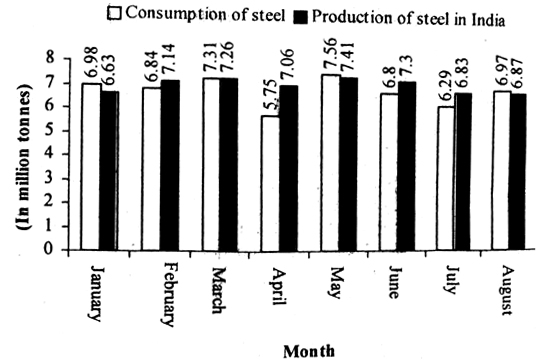### Data Interpretation Questions with Solution

Directions (1 to 5): Study the following information carefully and answer the questions given below:1). What is the difference between the amount of production of steel in India in February and May together and the consumption of steel in June and August? (In million tonnes).
a) 0.56
b) 1.78
c) 0.78
d) 1.56
e) None of these

2). The amount of consumption of steel in June is what per cent of the amount of total consumption of steel for the given months?
a) 12.48%
b) 13.76%
c) 11.48%
d) 13.43%
e) None of these

3). What is the average consumption of steel in India for the given months? (In million tonnes)
a) 5.5625
b) 6.8125
c) 8.6155
d) 7.5315
e) None of these

4). What is the ratio of the total consumption to the total production of steel for the given months?
a) 111 : 113
b) 109 : 111
c) 119 : 111
d) 109 : 113
e) None of these

5). What is the difference between the average consumption of steel for the given months and the average production of steel for the given months? (In tonnes)
a) 256250
b) 250000
c) 260000
d) 257500
e) None of these

Direction (Q. 6-10): Study the following pie-charts carefully to answer the questions that follow:

Percentage of employees in different departments in a company

6). What is the number of employees in Audit?
a) 350
b) 330
c) 450
d) 336
e) 216

7). What is the average number of all female employees in all departments?
a) 80.5
b) 92.5
c) 89.2
d) 91.3
e) 81.5

8). Number of girls in finance department is what percent of number of boys in Audit?
a) 70.5%
b) 60.5%
c) 51.5%
d) 55.5%
e) 62.5%

9). Number of female employees in Audit is approx what percent more than male in personnel?
a) 8%
b) 5%
c) 6%
d) 9%
e) 10%

10). What is the difference between Male of IT and operations together and girls of IT and HR together?
a) 64
b) 60
c) 150
d) 124
e) 134

1). Reqd difference
= (7.14 + 7.41) - (6.80 + 6.97)
= 14.55-13.77 = 0.78 tonnes

2). Total consumption of steel in June=6.8 million tonnes
Total consumption of steel over the given month = 6.98 + 6.84 + 7.31 + 5.75 + 7.56 + 6.8 + 6.29 + 6.97
= 54.5 million tones
Reqd % = (6.8/54.5) x100 = 12.48%

3). Reqd average
= (6.98 + 6.84 + 7.31+ 5.75 + 7.56 + 6.8 + 6.29 + 6.97)/8
=54.5/8 = 6.8125 million tones

4). Total consumption of steel over the given months = 6.98 + 6.84 + 7.31 + 5.75 +
7.56 + 6.8 + 6.29 + 6.97 = 54.5
Total production of steel over the given months = 6.63 + 7.14 + 7.26 + 7.06 + 7.41
+ 7.30 + 6.83 + 6.87 = 56.5
:. Reqd ratio = 54.5/56.5 = 109 : 113

5). Reqd average = (56.5 – 54.5)/8 million tones = 2/8 million tones= 250000 tonnes